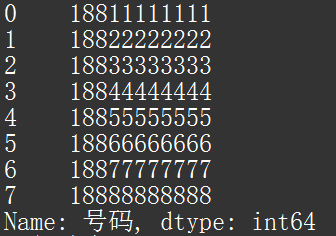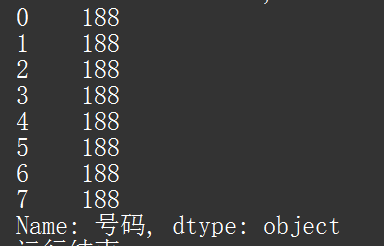# Excel 处理技巧 - 数据处理 （三）

### 一、字段抽取

`slice(start,stop)`

``````  start 表示开始位置
stop  表示结束位置
``````

``````df.[列名].str.slice(开始位置,结束位置)
````````````df = pd.read_excel(io='//mac/Home/Desktop/test/test.xlsx')
df['号码'] = df['号码'].astype(str)                 # 属性转成字符串格式
df1 = df['号码'].str.slice(0,3)
print(df1)
``````### 二、字段拆分

`split(sep,n,expand=False)`

``````sep     表示用于分割字符串的分隔符；
n       表示分割后新增的列数；
expand  表示 是否展开为数据框，默认False,返回的是Series
为True,返回的是DateFrame
````````````df = pd.read_excel(io='//mac/Home/Desktop/test/test.xlsx')
df['IP'] = df['IP'].astype(str)
df1 = df['IP'].str.split('.',4,True)
print(df1)
``````### 三、记录抽取

`df[condition]`

``````condition  表示过滤条件
````````````df = pd.read_excel(io='//mac/Home/Desktop/test/test.xlsx')
df1= df[df.英语.between(65,75)]
print(df1)
``````